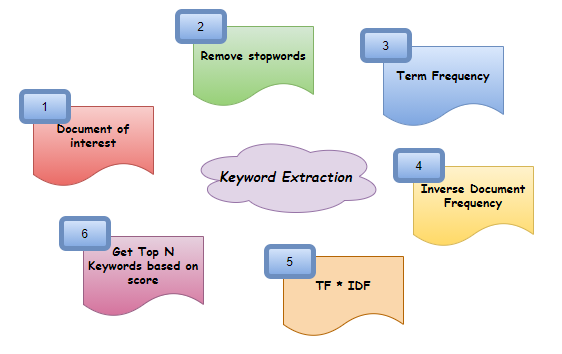Prachi Prakash — November 26, 2020

This article was published as a part of the Data Science Blogathon.

## IntroductionUnstructured data contains a plethora of information. It is like energy when harnessed, will create high value for its stakeholders. A lot of work is already being done in this area by various companies. There is no doubt that the unstructured data is noisy and significant work has to be done to clean, analyze, and make them meaningful to use. This article talks about an area which helps analyze large amounts of data by summarizing the content and identifying topics of interest – Keyword Extraction

## Keyword Extraction Overview

It is a text analysis technique. We can obtain important insights into the topic within a short span of time. It helps concise the text and obtain relevant keywords. It saves the time of going through the entire document. Example use-cases are finding topics of interest from a news article and identifying the problems based on customer reviews and so. One of the techniques used for Keyword Extraction is TF-IDF ( Term Frequency – Inverse Document Frequency )

## TF – IDF Overview

Term Frequency – How frequently a term occurs in a text. It is measured as the number of times a term t appears in the text / Total number of words in the document

Inverse Document Frequency – How important a word is in a document. It is measured as log(total number of sentences / Number of sentences with term t)

TF-IDF – Words’ importance is measure by this score. It is measured as TF * IDF

We will use the same concept and try to code it line by line using Python. We will take a smaller set of text documents and perform all the steps above. While higher concepts for keyword extraction are already in place in the market, this article is aimed at understanding the basic concept behind identifying word importance. Let’s get started!

## Implementation

#### 1. Import Packages

We need to tokenize to create word tokens, itemgetter to sort the dictionary, and math to perform log base e operation

```from nltk import tokenize
from operator import itemgetter
import math```

#### 2. Declare Variables

We will declare a string variable. It will be a placeholder for the sample text document

`doc = 'I am a graduate. I want to learn Python. I like learning Python. Python is easy. Python is interesting. Learning increases thinking. Everyone should invest time in learning'`

#### 3. Remove stopwords

Stopwords are the frequently occurring words that may not carry significance to our analysis. We can remove the using nltk library

```import nltk
from nltk.corpus import stopwords
from nltk.tokenize import word_tokenize
stop_words = set(stopwords.words('english'))```

#### 4. Find total words in the document

This will be required while calculating Term Frequency

```total_words = doc.split()
total_word_length = len(total_words)
print(total_word_length)```

#### 5. Find the total number of sentences

This will be required while calculating Inverse Document Frequency

```total_sentences = tokenize.sent_tokenize(doc)
total_sent_len = len(total_sentences)
print(total_sent_len)```

#### 6. Calculate TF for each word

We will begin by calculating the word count for each non-stop words and finally divide each element by the result of step 4

```tf_score = {}
for each_word in total_words:
each_word = each_word.replace('.','')
if each_word not in stop_words:
if each_word in tf_score:
tf_score[each_word] += 1
else:
tf_score[each_word] = 1

# Dividing by total_word_length for each dictionary element
tf_score.update((x, y/int(total_word_length)) for x, y in tf_score.items())
print(tf_score)```

#### 7. Function to check if the word is present in a sentence list

This method will be required when calculating IDF

```def check_sent(word, sentences):
final = [all([w in x for w in word]) for x in sentences]
sent_len = [sentences[i] for i in range(0, len(final)) if final[i]]
return int(len(sent_len))```

#### 8. Calculate IDF for each word

We will use the function in step 7 to iterate the non-stop word and store the result for Inverse Document Frequency

```idf_score = {}
for each_word in total_words:
each_word = each_word.replace('.','')
if each_word not in stop_words:
if each_word in idf_score:
idf_score[each_word] = check_sent(each_word, total_sentences)
else:
idf_score[each_word] = 1

# Performing a log and divide
idf_score.update((x, math.log(int(total_sent_len)/y)) for x, y in idf_score.items())

print(idf_score)```

#### 9. Calculate TF * IDF

Since the key of both the dictionary is the same, we can iterate one dictionary to get the keys and multiply the values of both

```tf_idf_score = {key: tf_score[key] * idf_score.get(key, 0) for key in tf_score.keys()}
print(tf_idf_score)```

#### 10. Create a function to get N important words in the document

```def get_top_n(dict_elem, n):
result = dict(sorted(dict_elem.items(), key = itemgetter(1), reverse = True)[:n])
return result```

#### 11. Get the top 5 words of significance

`print(get_top_n(tf_idf_score, 5))`

## Conclusion

So, this is one of the ways you can build your own keyword extractor in Python! The steps above can be summarized in a simple way as Document -> Remove stop words -> Find Term Frequency (TF) -> Find Inverse Document Frequency (IDF) -> Find TF*IDF -> Get top N Keywords. Do share your thoughts if this article was interesting or helped you in any way. Always open to improvements and suggestions. You can find the code on